The finafit package brings together the day-to-day functions we use to generate final results tables and plots when modelling.

I spent many years repeatedly manually copying results from R analyses and built these functions to automate our standard healthcare data workflow. It is particularly useful when undertaking a large study involving multiple different regression analyses. When combined with RMarkdown, the reporting becomes entirely automated. Its design follows Hadley Wickham’s tidy tool manifesto.

## Installation and Documentation

Development lives on GitHub.

You can install the finalfit development version from CRAN with:

install.packages("finalfit")

It is recommended that this package is used together with dplyr, which is a dependent.

Some of the functions require rstan and boot. These have been left as Suggests rather than Depends to avoid unnecessary installation. If needed, they can be installed in the normal way:

install.packages("rstan")
install.packages("boot")

To install off-line (or in a Safe Haven), download the zip file and use devtools::install_local().

## Main Features

### 1. Summarise variables/factors by a categorical variable

summary_factorlist() is a wrapper used to aggregate any number of explanatory variables by a single variable of interest. This is often “Table 1” of a published study. When categorical, the variable of interest can have a maximum of five levels. It uses Hmisc::summary.formula().

library(finalfit)
library(dplyr)

# Load example dataset, modified version of survival::colon
data(colon_s)

# Table 1 - Patient demographics by variable of interest ----
explanatory = c("age", "age.factor", "sex.factor", "obstruct.factor")
dependent = "perfor.factor" # Bowel perforation
colon_s %>%
summary_factorlist(dependent, explanatory,
knitr::kable(t1, row.names=FALSE, align=c("l", "l", "r", "r", "r"))
Dependent: Perforation No Yes p
Age (years) Mean (SD) 59.8 (11.9) 58.4 (13.3) 0.542
Age <40 years 68 (7.5) 2 (7.4) 1.000
40-59 years 334 (37.0) 10 (37.0)
60+ years 500 (55.4) 15 (55.6)
Sex Female 432 (47.9) 13 (48.1) 1.000
Male 470 (52.1) 14 (51.9)
Obstruction No 715 (81.2) 17 (63.0) 0.035
Yes 166 (18.8) 10 (37.0)

When exported to PDF: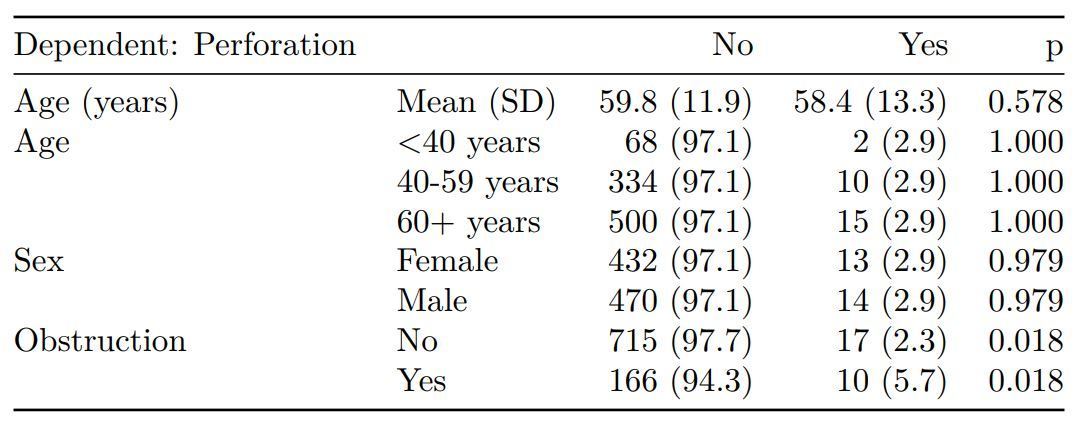See other options relating to inclusion of missing data, mean vs. median for continuous variables, column vs. row proportions, include a total column etc.

summary_factorlist() is also commonly used to summarise any number of variables by an outcome variable (say dead yes/no).

# Table 2 - 5 yr mortality ----
explanatory = c("age.factor", "sex.factor", "obstruct.factor")
dependent = 'mort_5yr'
colon_s %>%
summary_factorlist(dependent, explanatory,
knitr::kable(t2, row.names=FALSE, align=c("l", "l", "r", "r", "r"))
Dependent: Mortality 5 year Alive Died p
Age <40 years 31 (6.1) 36 (8.9) 0.020
40-59 years 208 (40.7) 131 (32.4)
60+ years 272 (53.2) 237 (58.7)
Sex Female 243 (47.6) 194 (48.0) 0.941
Male 268 (52.4) 210 (52.0)
Obstruction No 408 (82.1) 312 (78.6) 0.219
Yes 89 (17.9) 85 (21.4)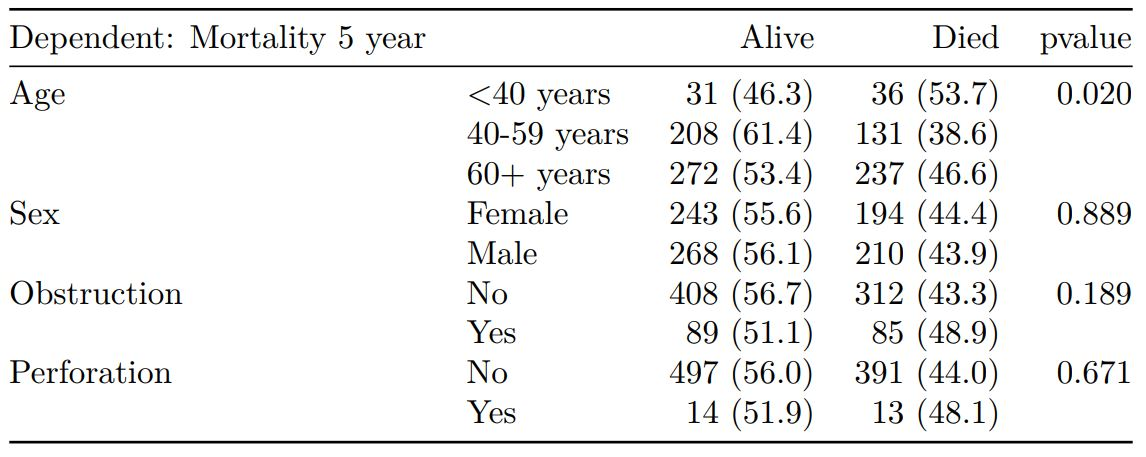Tables can be knitted to PDF, Word or html documents. We do this in RStudio from a .Rmd document.

### 2. Summarise regression model results in final table format

The second main feature is the ability to create final tables for linear lm(), logistic glm(), hierarchical logistic lme4::glmer() and Cox proportional hazards survival::coxph() regression models.

The finalfit() “all-in-one” function takes a single dependent variable with a vector of explanatory variable names (continuous or categorical variables) to produce a final table for publication including summary statistics, univariable and multivariable regression analyses. The first columns are those produced by summary_factorist(). The appropriate regression model is chosen on the basis of the dependent variable type and other arguments passed.

#### Logistic regression: glm()

Of the form: glm(depdendent ~ explanatory, family="binomial")

explanatory = c("age.factor", "sex.factor", "obstruct.factor", "perfor.factor")
dependent = 'mort_5yr'
colon_s %>%
finalfit(dependent, explanatory) -> t3
knitr::kable(t3, row.names=FALSE, align=c("l", "l", "r", "r", "r", "r"))
Dependent: Mortality 5 year Alive Died OR (univariable) OR (multivariable)
Age <40 years 31 (46.3) 36 (53.7) - -
40-59 years 208 (61.4) 131 (38.6) 0.54 (0.32-0.92, p=0.023) 0.57 (0.34-0.98, p=0.041)
60+ years 272 (53.4) 237 (46.6) 0.75 (0.45-1.25, p=0.270) 0.81 (0.48-1.36, p=0.426)
Sex Female 243 (55.6) 194 (44.4) - -
Male 268 (56.1) 210 (43.9) 0.98 (0.76-1.27, p=0.889) 0.98 (0.75-1.28, p=0.902)
Obstruction No 408 (56.7) 312 (43.3) - -
Yes 89 (51.1) 85 (48.9) 1.25 (0.90-1.74, p=0.189) 1.25 (0.90-1.76, p=0.186)
Perforation No 497 (56.0) 391 (44.0) - -
Yes 14 (51.9) 13 (48.1) 1.18 (0.54-2.55, p=0.672) 1.12 (0.51-2.44, p=0.770)

#### Logistic regression with reduced model: glm()

Where a multivariable model contains a subset of the variables included specified in the full univariable set, this can be specified.

explanatory = c("age.factor", "sex.factor", "obstruct.factor", "perfor.factor")
explanatory_multi = c("age.factor", "obstruct.factor")
dependent = 'mort_5yr'
colon_s %>%
finalfit(dependent, explanatory, explanatory_multi) -> t4
knitr::kable(t4, row.names=FALSE, align=c("l", "l", "r", "r", "r", "r"))
Dependent: Mortality 5 year Alive Died OR (univariable) OR (multivariable)
Age <40 years 31 (46.3) 36 (53.7) - -
40-59 years 208 (61.4) 131 (38.6) 0.54 (0.32-0.92, p=0.023) 0.57 (0.34-0.98, p=0.041)
60+ years 272 (53.4) 237 (46.6) 0.75 (0.45-1.25, p=0.270) 0.81 (0.48-1.36, p=0.424)
Sex Female 243 (55.6) 194 (44.4) - -
Male 268 (56.1) 210 (43.9) 0.98 (0.76-1.27, p=0.889) -
Obstruction No 408 (56.7) 312 (43.3) - -
Yes 89 (51.1) 85 (48.9) 1.25 (0.90-1.74, p=0.189) 1.26 (0.90-1.76, p=0.176)
Perforation No 497 (56.0) 391 (44.0) - -
Yes 14 (51.9) 13 (48.1) 1.18 (0.54-2.55, p=0.672) -

#### Mixed effects logistic regression: lme4::glmer()

Of the form: lme4::glmer(dependent ~ explanatory + (1 | random_effect), family="binomial")

Hierarchical/mixed effects/multilevel logistic regression models can be specified using the argument random_effect. At the moment it is just set up for random intercepts (i.e. (1 | random_effect), but in the future I’ll adjust this to accommodate random gradients if needed (i.e. (variable1 | variable2).

explanatory = c("age.factor", "sex.factor", "obstruct.factor", "perfor.factor")
explanatory_multi = c("age.factor", "obstruct.factor")
random_effect = "hospital"
dependent = 'mort_5yr'
colon_s %>%
finalfit(dependent, explanatory, explanatory_multi, random_effect) -> t5
knitr::kable(t5, row.names=FALSE, align=c("l", "l", "r", "r", "r", "r"))
Dependent: Mortality 5 year Alive Died OR (univariable) OR (multilevel)
Age <40 years 31 (46.3) 36 (53.7) - -
40-59 years 208 (61.4) 131 (38.6) 0.54 (0.32-0.92, p=0.023) 0.73 (0.38-1.40, p=0.342)
60+ years 272 (53.4) 237 (46.6) 0.75 (0.45-1.25, p=0.270) 1.01 (0.53-1.90, p=0.984)
Sex Female 243 (55.6) 194 (44.4) - -
Male 268 (56.1) 210 (43.9) 0.98 (0.76-1.27, p=0.889) -
Obstruction No 408 (56.7) 312 (43.3) - -
Yes 89 (51.1) 85 (48.9) 1.25 (0.90-1.74, p=0.189) 1.24 (0.83-1.85, p=0.292)
Perforation No 497 (56.0) 391 (44.0) - -
Yes 14 (51.9) 13 (48.1) 1.18 (0.54-2.55, p=0.672) -

#### Cox proportional hazards: survival::coxph()

Of the form: survival::coxph(dependent ~ explanatory)

explanatory = c("age.factor", "sex.factor", "obstruct.factor", "perfor.factor")
dependent = "Surv(time, status)"
colon_s %>%
finalfit(dependent, explanatory) -> t6
knitr::kable(t6, row.names=FALSE, align=c("l", "l", "r", "r", "r", "r"))
Dependent: Surv(time, status) all HR (univariable) HR (multivariable)
Age <40 years 70 (7.5) - -
40-59 years 344 (37.0) 0.76 (0.53-1.09, p=0.132) 0.79 (0.55-1.13, p=0.196)
60+ years 515 (55.4) 0.93 (0.66-1.31, p=0.668) 0.98 (0.69-1.40, p=0.926)
Sex Female 445 (47.9) - -
Male 484 (52.1) 1.01 (0.84-1.22, p=0.888) 1.02 (0.85-1.23, p=0.812)
Obstruction No 732 (80.6) - -
Yes 176 (19.4) 1.29 (1.03-1.62, p=0.028) 1.30 (1.03-1.64, p=0.026)
Perforation No 902 (97.1) - -
Yes 27 (2.9) 1.17 (0.70-1.95, p=0.556) 1.08 (0.64-1.81, p=0.785)

#### Add common model metrics to output

metrics=TRUE provides common model metrics. The output is a list of two dataframes. Note chunk specification for output below.

explanatory = c("age.factor", "sex.factor",
"obstruct.factor", "perfor.factor")
dependent = 'mort_5yr'
colon_s %>%
finalfit(dependent, explanatory, metrics=TRUE) -> t7
knitr::kable(t7[], row.names=FALSE, align=c("l", "l", "r", "r", "r", "r"))
Dependent: Mortality 5 year Alive Died OR (univariable) OR (multivariable)
Age <40 years 31 (46.3) 36 (53.7) - -
40-59 years 208 (61.4) 131 (38.6) 0.54 (0.32-0.92, p=0.023) 0.57 (0.34-0.98, p=0.041)
60+ years 272 (53.4) 237 (46.6) 0.75 (0.45-1.25, p=0.270) 0.81 (0.48-1.36, p=0.426)
Sex Female 243 (55.6) 194 (44.4) - -
Male 268 (56.1) 210 (43.9) 0.98 (0.76-1.27, p=0.889) 0.98 (0.75-1.28, p=0.902)
Obstruction No 408 (56.7) 312 (43.3) - -
Yes 89 (51.1) 85 (48.9) 1.25 (0.90-1.74, p=0.189) 1.25 (0.90-1.76, p=0.186)
Perforation No 497 (56.0) 391 (44.0) - -
Yes 14 (51.9) 13 (48.1) 1.18 (0.54-2.55, p=0.672) 1.12 (0.51-2.44, p=0.770)
knitr::kable(t7[], row.names=FALSE, col.names="")
 Number in dataframe = 929, Number in model = 894, Missing = 35, AIC = 1230.7, C-statistic = 0.56, H&L = Chi-sq(8) 5.69 (p=0.682)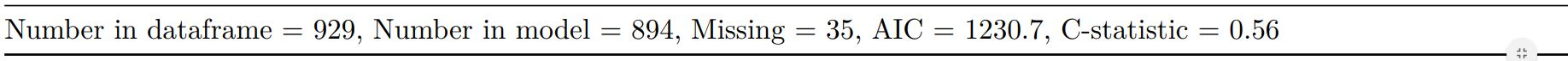#### Combine multiple models into single table

Rather than going all-in-one, any number of subset models can be manually added on to a summary_factorlist() table using finalfit_merge(). This is particularly useful when models take a long-time to run or are complicated.

Note the requirement for fit_id=TRUE in summary_factorlist(). fit2df extracts, condenses, and add metrics to supported models.

explanatory = c("age.factor", "sex.factor", "obstruct.factor", "perfor.factor")
explanatory_multi = c("age.factor", "obstruct.factor")
random_effect = "hospital"
dependent = 'mort_5yr'

# Separate tables
colon_s %>%
summary_factorlist(dependent,
explanatory, fit_id=TRUE) -> example.summary

colon_s %>%
glmuni(dependent, explanatory) %>%
fit2df(estimate_suffix=" (univariable)") -> example.univariable

colon_s %>%
glmmulti(dependent, explanatory) %>%
fit2df(estimate_suffix=" (multivariable)") -> example.multivariable

colon_s %>%
glmmixed(dependent, explanatory, random_effect) %>%
fit2df(estimate_suffix=" (multilevel)") -> example.multilevel

# Pipe together
example.summary %>%
finalfit_merge(example.univariable) %>%
finalfit_merge(example.multivariable) %>%
finalfit_merge(example.multilevel, last_merge = TRUE) %>%
dependent_label(colon_s, dependent, prefix="") -> t8 # place dependent variable label
knitr::kable(t8, row.names=FALSE, align=c("l", "l", "r", "r", "r", "r", "r"))
Mortality 5 year Alive Died OR (univariable) OR (multivariable) OR (multilevel)
Age <40 years 31 (6.1) 36 (8.9) - - -
40-59 years 208 (40.7) 131 (32.4) 0.54 (0.32-0.92, p=0.023) 0.57 (0.34-0.98, p=0.041) 0.75 (0.39-1.44, p=0.382)
60+ years 272 (53.2) 237 (58.7) 0.75 (0.45-1.25, p=0.270) 0.81 (0.48-1.36, p=0.426) 1.03 (0.55-1.96, p=0.916)
Sex Female 243 (47.6) 194 (48.0) - - -
Male 268 (52.4) 210 (52.0) 0.98 (0.76-1.27, p=0.889) 0.98 (0.75-1.28, p=0.902) 0.80 (0.58-1.11, p=0.180)
Obstruction No 408 (82.1) 312 (78.6) - - -
Yes 89 (17.9) 85 (21.4) 1.25 (0.90-1.74, p=0.189) 1.25 (0.90-1.76, p=0.186) 1.23 (0.82-1.83, p=0.320)
Perforation No 497 (97.3) 391 (96.8) - - -
Yes 14 (2.7) 13 (3.2) 1.18 (0.54-2.55, p=0.672) 1.12 (0.51-2.44, p=0.770) 1.03 (0.43-2.51, p=0.940)

#### Bayesian logistic regression: with stan

Our own particular rstan models are supported and will be documented in the future. Broadly, if you are running (hierarchical) logistic regression models in Stan with coefficients specified as a vector labelled beta, then fit2df() will work directly on the stanfit object in a similar manner to if it was a glm or glmerMod object.

### 3. Summarise regression model results in plot

Models can be summarized with odds ratio/hazard ratio plots using or_plot, hr_plot and surv_plot.

#### OR plot

explanatory = c("age.factor", "sex.factor", "obstruct.factor", "perfor.factor")
dependent = 'mort_5yr'
colon_s %>%
or_plot(dependent, explanatory)
# Previously fitted models (glmmulti() or # glmmixed()) can be provided directly to glmfit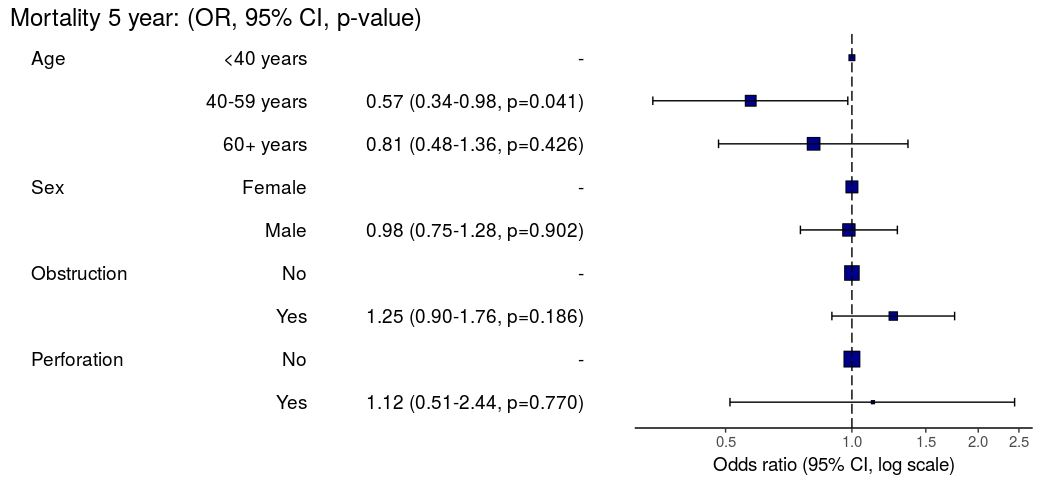#### HR plot

explanatory = c("age.factor", "sex.factor", "obstruct.factor", "perfor.factor")
dependent = "Surv(time, status)"
colon_s %>%
hr_plot(dependent, explanatory, dependent_label = "Survival")
# Previously fitted models (coxphmulti) can be provided directly using coxfit#### Kaplan-Meier survival plots

KM plots can be produced using the library(survminer)

explanatory = c("perfor.factor")
dependent = "Surv(time, status)"
colon_s %>%
surv_plot(dependent, explanatory,
xlab="Time (days)", pval=TRUE, legend="none")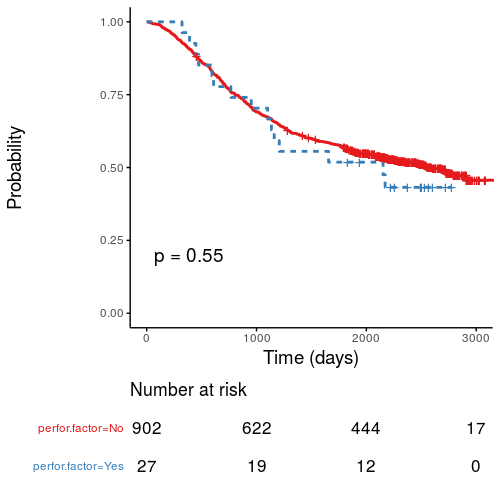Use ff_label() to assign labels to variables for tables and plots.

colon_s %>%
mutate(
ff_label(age.factor, "Age (years)")
)

Export dataframe tables directly or to R Markdown knitr::kable().

Note wrapper missing_pattern() is also useful. Wraps mice::md.pattern.

colon_s %>%
missing_pattern(dependent, explanatory)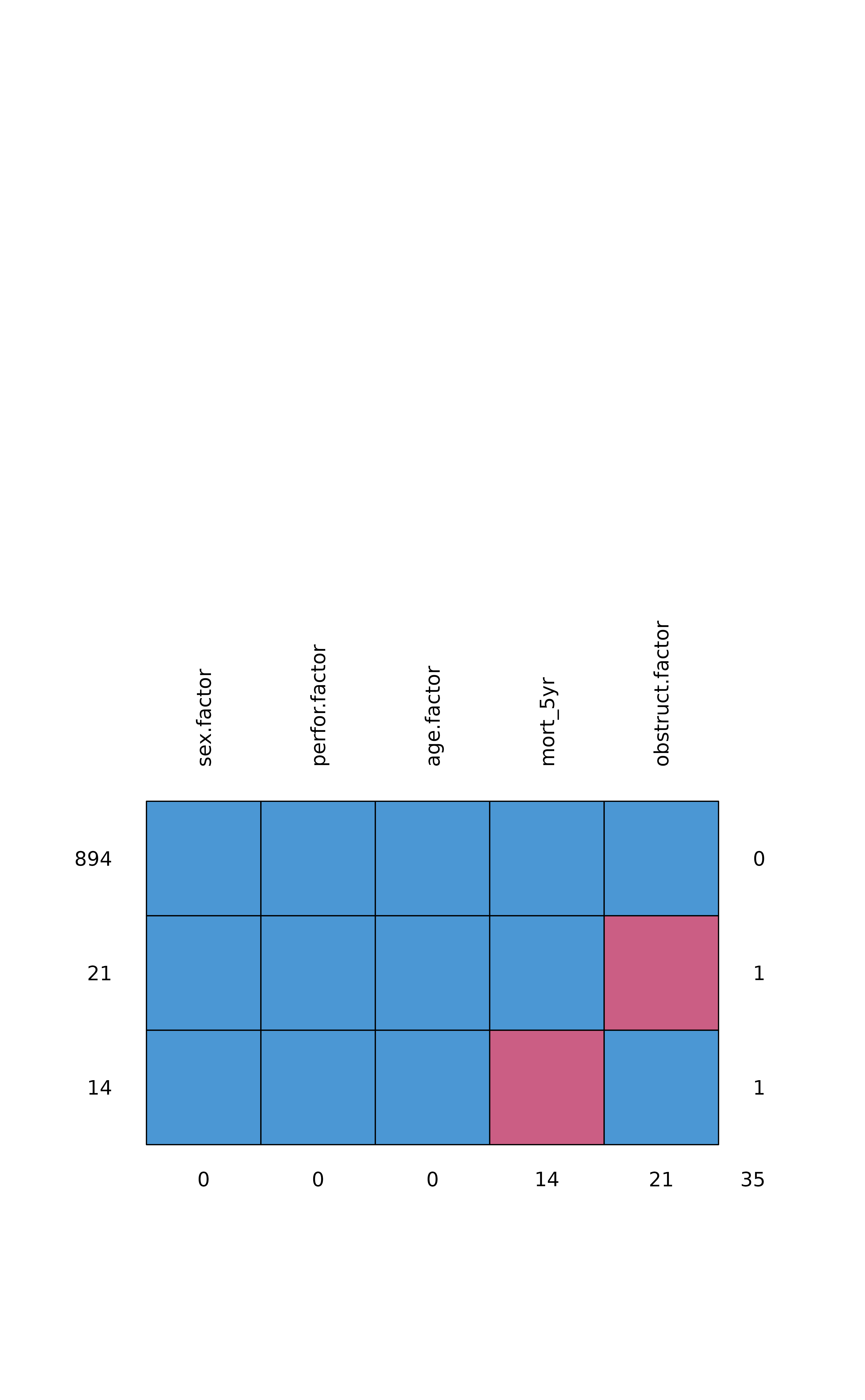#>     sex.factor perfor.factor age.factor mort_5yr obstruct.factor
#> 894          1             1          1        1               1  0
#> 21           1             1          1        1               0  1
#> 14           1             1          1        0               1  1
#>              0             0          0       14              21 35

Development will be on-going, but any input appreciated.